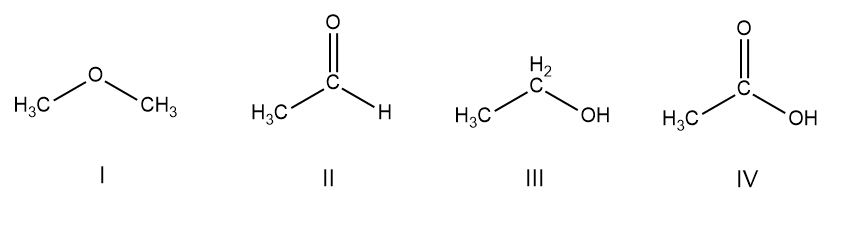# Problem: Rank the following compounds in order of decreasing acidity, putting the most acidic first. why?                 CH3OCH3          CH3CHO         CH3CH2OH          CH3COOH                         I                        II                        III                            IV

###### FREE Expert Solution

We are asked to rank the following compounds in order of decreasing acidity.Find the hydrogen and analyze where it is attached.

I) Ether → O-C-H → C is lower in electronegativity than O.

II) Aldehyde → O=C-H → resonance due to pi bond.

III) Alcohol → C-O-H → Acidic due to electronegative O

IV) Carboxylic acid → O=C-O-H →   Acidic due to electronegative O and resonance due to pi bond.###### Problem Details

Rank the following compounds in order of decreasing acidity, putting the most acidic first. why?

CH3OCH3          CH3CHO         CH3CH2OH          CH3COOH

I                        II                        III                            IV